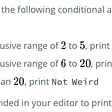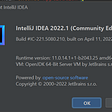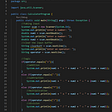# Strobogrammatic Number II — Day 91(Python)

Today’s question is a medium-tagged question on leetcode. Let us look into the problem statement.

247. Strobogrammatic Number II

Given an integer `n`, return all the strobogrammatic numbers that are of length `n`. You may return the answer in any order.

A strobogrammatic number is a number that looks the same when rotated `180` degrees (looked at upside down).

Example 1:

`Input: n = 2Output: ["11","69","88","96"]`

Example 2:

`Input: n = 1Output: ["0","1","8"]`

Constraints:

• `1 <= n <= 14`

Before we start with solving this problem, let us understand what strobogrammatic numbers are. A strobogrammatic number is a number that looks the same when rotated 180 degrees. So which are the strobogrammatic number? 0, 1, 8 are the digits that are strobogrammatic. 6 and 9, when used together, form strobogrammatic numbers.

We have “n” which is the number of digits in the number that we form that has to be checked if they form a strobogrammatic number.

One way to solve this problem is by creating a dictionary that stores each number that can form a strobogrammatic number and its corresponding values.

`char_list = ["0", "1", "6", "8", "9"]strobogrammatic_dict = {"0":"0","1":"1","6":"9","8":"8","9":"6"}`

We will use 2 strings; one to save the number formed another to save its possible rotated number. When the number of digits is equal to n, if the number formed is equal to the number saved in a rotated number, we will save it as part of the result.

`class StrobogrammaticFinder:  def findStrobogrammatic(self, n: int) -> List[str]:      output = []      char_list = ["0", "1", "6", "8", "9"]      strobo_dict = {"0":"0","1":"1","6":"9","8":"8","9":"6"}      def dfs(tmp, st_tmp):          if len(tmp) == n:             if tmp == st_tmp[::-1]:                if str(int(tmp)) == tmp:                   output.append(tmp)             return          for i in range(len(char_list)):              dfs(tmp+char_list[i],st_tmp+strobo_dict[char_list[i]])                 dfs("","")      return(output)`

# Complexity analysis.

Time Complexity

For all n digits, we have 5 digits to choose from. Hence time complexity is O(5^N).

Space Complexity

Since we are recursively calling the dfs function, and internally recursive calls are stored in form of a stack. The space complexity is O(5^N).

When we run this code on leetcode, we will likely hit the time limit exceeded error. Is there a way to reduce the number of calls?

Do we have to find numbers for all the n places? Can we try to find only until the half portion? If we find just until the half position, we can rotate this number and set it for the other half. Or even better, we start from both the extreme positions and move inwards.

Let us see how we can code this.

`class StrobogrammaticFinder:  def findStrobogrammatic(self, n: int) -> List[str]:        output = []        # Create a dictionary        strobo_dict = {"0":"0","1":"1","6":"9","8":"8","9":"6"}        start = 0        end = n-1        #Create a list of size n initialised with None        result = [None]*n        self.dfs(start, end, result, strobo_dict, output)        return output      def dfs(self, start, end, result, strobo_dict, output):        if start > end:            output.append(''.join(result))            return        #For each number in the dictionary        for each_num in strobo_dict:            #if it is single digit number            if start!= end and each_num == '0' and start == 0:                continue            # if we have reached the middle            if start == end and each_num in ('6','9'):                continue                            result[start], result[end] = each_num, strobo_dict[each_num]            self.dfs(start+1, end-1, result, strobo_dict, output)`

# Complexity analysis.

Time Complexity

For all n digits, we have 5 digits to chose from, but in the above code, we start from both the extreme positions and move inwards. Hence time complexity is O(5^(N/2)).

Space Complexity

Since we are recursively calling the dfs function, and internally recursive calls are stored in form of a stack. The space complexity is O(5^(N/2)).

--

--

--

## More from Nerd For Tech

NFT is an Educational Media House. Our mission is to bring the invaluable knowledge and experiences of experts from all over the world to the novice. To know more about us, visit https://www.nerdfortech.org/.

## Rooting & Custom Roms— Part 1## Auditing with Lighthouse - Quality Assurance Checkpoint for WebApp!## Starting a New ASP.NET Core 2.2 C# Project with Visual Studio 2017## Keep your secrets safe with Azure Key Vault in Python## What is an Autonomous Wireless Network?## Meet the Power Apps Champions## Weird Numbers in Java## Annamariya Tharayil

Software Engineer. Find me @ www.linkedin.com/in/annamariya-jt

## [Solved] Error: JavaFX runtime components are missing, and are required to run this application## The Art Of Programming #9